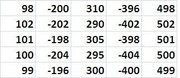• NEW! FREE Beat The GMAT QuizzesHundreds of Questions Highly Detailed Reporting Expert Explanations
• 7 CATs FREE!
If you earn 100 Forum Points

Engage in the Beat The GMAT forums to earn
100 points for $49 worth of Veritas practice GMATs FREEVERITAS PRACTICE GMAT EXAMS Earn 10 Points Per Post Earn 10 Points Per Thanks Earn 10 Points Per Upvote ## What is the sum of all of the integers in the chart above? tagged by: BTGmoderatorLU ##### This topic has 3 expert replies and 0 member replies ### Top Member ## What is the sum of all of the integers in the chart above?## Timer 00:00 ## Your Answer A B C D E ## Global Stats Difficult Source: Manhattan GMATWhat is the sum of all of the integers in the chart above? A. 0 B. 300 C. 500 D. 1500 E. 6500 The OA is D ### GMAT/MBA Expert GMAT InstructorJoined 02 Jun 2008 Posted: 2524 messages Followed by: 352 members Upvotes: 1090 GMAT Score: 780 In each column, if we list the numbers in increasing order, we get an equally spaced list with a multiple of 100 in the middle. So, for example, the second column contains the numbers: -204, -202, -200, -198, -196 The sum of an equally spaced list is just equal to the average (which, in an equally spaced list, is the median) of the list times the number of things in the list, so the five numbers above add to (5)(-200). Doing that for each column, we get the overall sum: (5)(100) + (5)(-200) + (5)(300) + (5)(-400) + (5)(500) = (5) (100-200+300-400+500) = (5)(300) = 1500 _________________ If you are looking for online GMAT math tutoring, or if you are interested in buying my advanced Quant books and problem sets, please contact me at ianstewartgmat at gmail.com ### GMAT/MBA Expert GMAT InstructorJoined 08 Dec 2008 Posted: 13033 messages Followed by: 1251 members Upvotes: 5254 GMAT Score: 770 BTGmoderatorLU wrote: Source: Manhattan GMATWhat is the sum of all of the integers in the chart above? A. 0 B. 300 C. 500 D. 1500 E. 6500 The OA is D Always check the answer choices BEFORE solving the question!! Since the answer choices are nicely spread apart, we can be quite aggressive in our estimation Let's add the 5 numbers in each COLUMN COLUMN 1: Sum ≈500 COLUMN 2: Sum ≈-1000 COLUMN 3: Sum ≈1500 COLUMN 4: Sum ≈-2000 COLUMN 5: Sum ≈2500 TOTAL SUM ≈500 + (-1000) + 1500 + (-2000) + 2500 ≈1500 Answer: D Cheers, Brent _________________ Brent Hanneson – Creator of GMATPrepNow.com Use my video course along withSign up for free Question of the Day emails And check out all of these free resources GMAT Prep Now's comprehensive video course can be used in conjunction with Beat The GMAT’s FREE 60-Day Study Guide and reach your target score in 2 months! ### GMAT/MBA Expert GMAT InstructorJoined 25 Apr 2015 Posted: 2950 messages Followed by: 19 members Upvotes: 43 BTGmoderatorLU wrote: Source: Manhattan GMATWhat is the sum of all of the integers in the chart above? A. 0 B. 300 C. 500 D. 1500 E. 6500 The OA is D We don’t have actually add up all the numbers; we can just approximate the sum. Let’s add the numbers column by column. We see that the 5 numbers in the first column are each about 100, so the sum is approximately 500. The 5 numbers in the second column are each about -200, so the sum is approximately -1000. The 5 numbers in the third column are each about 300, so the sum is approximately 1500. The 5 numbers in the fourth column are each about -400, so the sum is approximately -2000. Lastly, the 5 numbers in the fifth column are each about 500, so the sum is approximately 2500. Therefore, the sum of all 25 numbers is approximately 500 + (-1000) + 1500 + (-2000) + 2500 = 4500 - 3000 = 1500 Answer: D. •Free Trial & Practice Exam BEAT THE GMAT EXCLUSIVE Available with Beat the GMAT members only code •Get 300+ Practice Questions 25 Video lessons and 6 Webinars for FREE Available with Beat the GMAT members only code •5 Day FREE Trial Study Smarter, Not Harder Available with Beat the GMAT members only code •Magoosh Study with Magoosh GMAT prep Available with Beat the GMAT members only code •1 Hour Free BEAT THE GMAT EXCLUSIVE Available with Beat the GMAT members only code •Free Veritas GMAT Class Experience Lesson 1 Live Free Available with Beat the GMAT members only code •Award-winning private GMAT tutoring Register now and save up to$200

Available with Beat the GMAT members only code

•5-Day Free Trial
5-day free, full-access trial TTP Quant

Available with Beat the GMAT members only code

•Free Practice Test & Review
How would you score if you took the GMAT

Available with Beat the GMAT members only code

•FREE GMAT Exam
Know how you'd score today for \$0

Available with Beat the GMAT members only code

### Top First Responders*

1Scott@TargetTestPrep 40 first replies
2Brent@GMATPrepNow 40 first replies
3Ian Stewart 37 first replies
4Jay@ManhattanReview 28 first replies
5GMATGuruNY 26 first replies
* Only counts replies to topics started in last 30 days
See More Top Beat The GMAT Members

### Most Active Experts

1Scott@TargetTestPrep

Target Test Prep

159 posts
2Max@Math Revolution

Math Revolution

84 posts
3Brent@GMATPrepNow

GMAT Prep Now Teacher

59 posts
4Ian Stewart

GMATiX Teacher

46 posts
5GMATGuruNY

The Princeton Review Teacher

40 posts
See More Top Beat The GMAT Experts